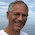## torsdag 28 maj 2015

### Physics as Analog Finite Precision Computation vs Physics as Statistics

I am exploring an approach to physics as "analog finite precision computation" to be be compared with classical physics as "analog infinite precision physics" and modern physics as "physics of dice games" or "statistical physics".

The step from classical to modern physics was forced upon physicists starting in the mid 19th century when it became clear that the 2nd law of thermodynamics could not be found in classical infinite precision physics of irreversible systems. The way to achieve irreversibility was to assume that atoms play dice games with the outcome of a throw of a dice inherently irreversible: To "unthrow" a dice was (correctly) understood to be impossible and thus irreversibility was introduced and the paralysis of reversible classical physics was broken. So far so good.

But the fix came with severe side effects as real physics independent of human observation was replaced by statistical physics representing "human understanding", as if the world goes around just because some physicist  is making observations and claim them to be understandable. Einstein and Schrödinger could never be convinced that atoms play dice, despite major pressure from the physics community.

The unfortunate result of this collapse of rationality of deterministic physics, has led modern physics into wildly speculative physics of strings and multiverse, which nobody can understand.

But there is a milder way of introducing irreversibility into classical reversible physics, and that is to view physics as analog computation with finite precision instead of infinite precision.

This connects directly to a computer operating with finite decimal expansion of real numbers as a necessary restriction of infinite decimal expansion, in order to allow computations to be performed in finite time: In order to make the world go around, and it does go around, and thus not come into a halt, physical processes cannot be realised with infinite precision and thus finite precision computation is a must in a world that goes around. It is thus necessary, but it is also sufficient to introduce irreversibility into classical reversible physics.

Finite precision computation thus solves the main problem which motivated the introduction of statistical physics, but in a much more gentle way and without the severe side effects of full-blown statistics based on dice games.

Finite precision computational physics is represented by the modern computer, while statistical physics would correspond to a "dice computer" throwing a dice in every step of decision, just like the "dice man" created by the pseudonym Luke Rhinehart. The life of the "dice man" turned into misery, which can be compared with (reasonably) successful ordinary (reasonably controlled) life under finite precision, without a dice but with constant pressure to go onto the next day.

So if you want to compare finite precision analog physics to modern statistical physics, make the thought experiment of comparing your usual finite precision computer, which you use to your advantage, to a "dice computer" which would be completely unpredicatable. This is the comparison between an experienced computer wiz often getting reliable results, to a totally inexperienced user pushing the keys randomely and getting garbage.

Or make the comparison of getting married to a person which follows a principle of "finite precision" to a person like the "dice man" who is completely unpredictable. What would you prefer?

Few ideas can change your view in the same way as "physics as analog finite precision computation". Try it!

#### 2 kommentarer:

1.As long as I can remember I have always been wondering about when something goes from being infinitely small to being zero. In mathematics it is not a problem, but in physics and the real world I live in, it is definitely a case. Therefore I find your approach to physics by viewing the world as finite precision analog computation which definitely can be simulated by finite precision digital computation, very appealing and natural.

I look forward to future blog posts on the topic.

2.Thanks Christopher, we seem to share views so let us keep contact.